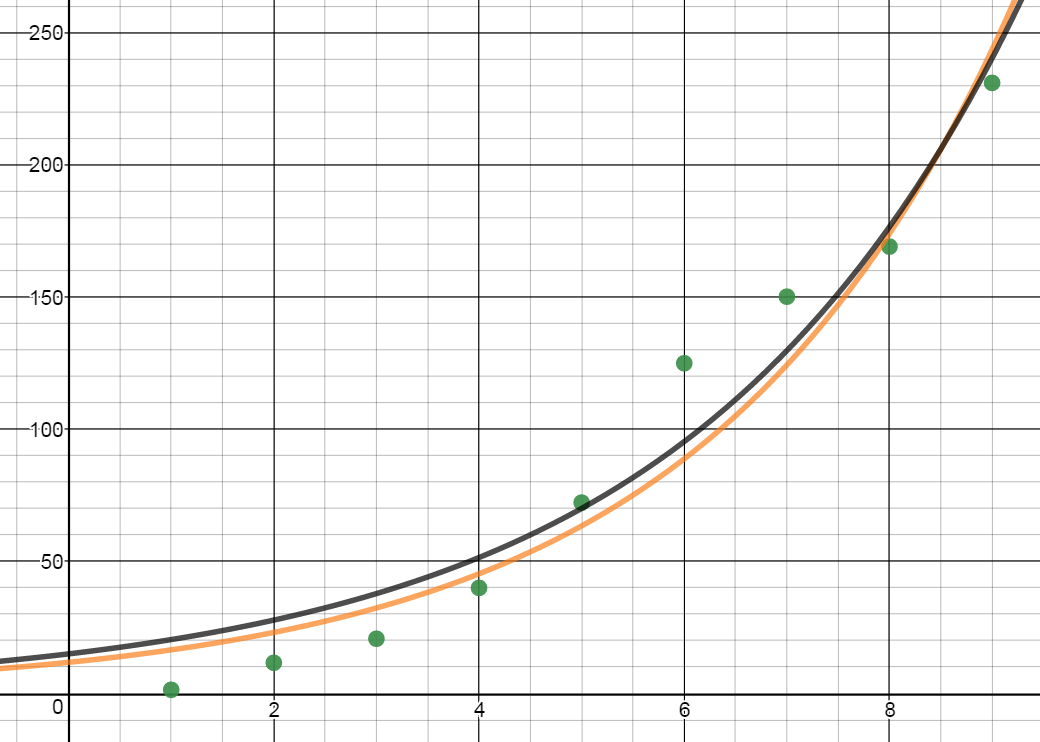# iPhone sales

/Tag:iPhone sales
•Equations from Data
Gallery

## Equations from Data

When businesses, governments and other policy makers prepare to make decisions, they often look at data to find trends. In this activity, we will use data and a graphing calculator, Desmos, or Geogebra to find an equation that best fits the data points. Statista has Global Apple iPod sales (in million units). Plot the data [...]

By | 2018-09-24T20:39:28+00:00 September 25th, 2016|Graphing Calculator, PreCalculus, Statistics|0 Comments

## Combinations of Functions and Composite Functions

Composite functions are a combination of two functions. The traditional math course introduces them like this: Let f and g be functions with overlapping domains. Then for all x common to both domains: (f + g)(x) = f(x) + g(x) (f - g)(x) = f(x) - g(x) (fg)(x) = f(x) · g(x) (f/g)(x) = f(x) / [...]

By | 2017-11-13T22:02:00+00:00 August 26th, 2015|PreCalculus, Statistics|0 Comments